# GSEB Solutions Class 8 Maths Chapter 11 Mensuration Ex 11.2

Gujarat Board GSEB Textbook Solutions Class 8 Maths Chapter 11 Mensuration Ex 11.2 Textbook Questions and Answers.

## Gujarat Board Textbook Solutions Class 8 Maths Chapter 11 Mensuration Ex 11.2

Question 1.
The shape of the top surface of a table is a trapezium. Find its area if its parallel sides are 1 m and 1.2 m and perpendicular distance between them is 0.8 m?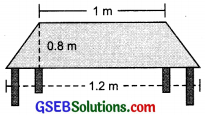Solution:
Area of a trapezium = $$\frac{1}{2}$$ × [Sum of parallel sides] × [Perpendicular distance between the parallel sides]
∴ Area of the table = $$\frac{1}{2}$$ [1.2 + 1] × 0.08 m2
= $$\frac{1}{2}$$ × 2.2 × 0.8 m2
= $$\frac{1}{2}$$ × $$\frac{22}{10}$$ × $$\frac{8}{10}$$ m2Question 2.
The area of a trapezium is 34 cm2 and the length of one of the parallel sides is 10 cm and its height is 4 cm. Find the length of the other parallel side?
Solution:
Let the length of other side be x cm
Area of the trapezium = $$\frac{1}{2}$$ × [Sum of parallel sides ] × [Height]
34 cm2 = $$\frac{1}{2}$$ × [10 + x] × 4 cm2
or $$\frac{2×34}{4}$$ cm2 = 10 + x
or 10 + x = 17 ⇒ x = 17 – 10 = 7 cm.
Hence, the length of other side = 7 cm.

Question 3.
Length of the fence of a trapezium shaped field ABCD is 120 m. If BC = 48 m, CD = 17 m and AD = 40 m, find the area of this field. Side AB is perpendicular to the parallel sides AD and BC?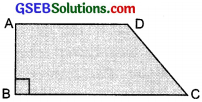Solution:
Length of the fence = Perimeter
∴ AB + BC + CD + DA = 120
or AB + 48 + 17 + 40 = 120
or AB + 105 = 120
or AB = 120 – 105 = 15 m

∵ Area of a trapezium
= $$\frac{1}{2}$$ × [Sum of parallel sides] × Height
= $$\frac{1}{2}$$ × (AD + BC) × AB
= $$\frac{1}{2}$$ × (40 + 48) × 15 m2
= $$\frac{1}{2}$$ × 88 × 15 m2 = 660 m2Question 4.
The diagonal of a quadrilateral shaped field is 24 m and the perpendiculars dropped on it from the remaining opposite vertices are 8 m and 13 m. Find the area of the field?
Solution: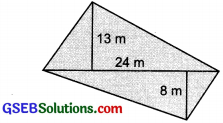= $$\frac{1}{2}$$ × Diagonal × [Sum of the perpendiculars on the diagonal from opposite vertices]
= $$\frac{1}{2}$$ × 24 × (8 + 13) m2
= $$\frac{1}{2}$$ × 24 × 21 m2 = 12 × 21 m2 = 252 m2

Question 5.
The diagonals of a rhombus are 7.5 cm and 12 cm. Find its area?
Solution:
Area of the rhombus = $$\frac{1}{2}$$ × Product of diagonals
= $$\frac{1}{2}$$ × 7.5 cm × 12 cm = $$\frac{1}{2}$$ × $$\frac{75}{10}$$ × $$\frac{12}{1}$$
= 15 × 3 cm2 = 45 cm2

Question 6.
Find the area of a rhombus whose side is 6 cm and whose altitude is 4 cm. If one of its diagonals is 8 cm long, find the length of the other diagonal?
Solution:
A rhombus is a parallelogram.
Area of a parallelogram = Base × Height
∴ Area of the rhombus = Base × Height
= 6 × 4 cm2
= 24 cm2
Let the other diagonal be d.
∴ Area of the rhombus = $$\frac{1}{2}$$ × 8 × d = 4d
[∵ One of the diagonals = 8 cm]
or 4d = 24
or d = $$\frac{24}{4}$$ = 6 cm
Thus, the required other diagonal is 6cm.Question 7.
The floor of a building consists of 3000 tiles which are rhombus shaped and each of its diagonals are 45 cm and 30 cm in length. Find the total cost of polishing the floor, if the cost per m2 is ₹ 4?
Solution:
Tiles are rhombus shaped, having d1 = 45 cm and d2 = 30 cm.
∴ Area of a tile (rhombus) = $$\frac{1}{2}$$ × d1 × d2
= $$\frac{1}{2}$$ × 45 × 30 cm2 = 45 × 15 cm2 = 675 cm2
Total number of tiles = 3000
∴ Area of the floor = 675 × 3000 cm2
= 2025000 cm2
= $$\frac{2025000}{100 \times 100}$$ m2 [∵ 1 m2 = 100 × 100 cm2]
= $$\frac{2025}{10}$$ m2
Cost of polishing the floor = ₹ 4 × $$\frac{2025}{10}$$
= ₹ 2 × 405 = ₹ 810

Question 8.
Mohan wants to buy a trapezium shaped field. Its side along the river field. Its side along the river is parallel to and twice the side along road. If the area of this field is 10500 m2 and the perpendicular distance between the two parallel sides is 100 m, find the length of the side along the river?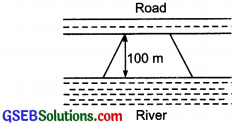Solution:
Let the length of the side along the river be x m
∴ Other side (parallel to the road) = $$\frac{1}{2}$$ x m
∴ Area of the trapezium shaped field
= $$\frac{1}{2}$$ [x + $$\frac{1}{2}$$ x] × 100 m2
= $$\frac{1}{2}$$ ($$\frac{3x}{2}$$) × 100m2 = 75x m2
But area of the field = 10500 m2
or x = $$\frac{10500}{75}$$ = 140 m
Thus, length of the side along the river = 140 mQuestion 9.
Top surface of a raised platform is in the shape of a regular octagon as shown in the figure. Find the area of the octagonal surface?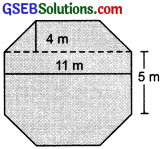Solution:
This regular octagon can be into two trapeziums (each having height 4 m and parallel sides are 11 m and 5 m) and a rectangular part with length 11 m and breadth 5 m.
Area of a trapezium = $$\frac{1}{2}$$ [11m + 5m] × 4m
= $$\frac{1}{2}$$ × 16 × 4 m2 = 32 m2
∴ Area of both the trapeziums = 2 × 32 m2 = 64m2
Now, Area of the rectangular part = l × b
= 11 m × 5 m = 55 m2
∴ Area of the regular octagon
= 64m2 + 55m2 = 119m2

Question 10.
There is a pentagonal shaped park as shown in the figure. For finding its area Jyoti and Kavita divided it in two dfferent ways?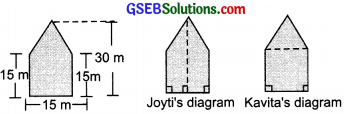Find the area of this park using both ways. Can you suggest some other way of finding its area?
Solution:
Jyoti’s diagram:
The given shape is splitted into two congruent trapeziums.
Area of one trapezium = $$\frac{1}{2}$$ × [15 + 30] × $$\frac{15}{2}$$m2
= $$\frac{1}{2}$$ × 45 × $$\frac{15}{2}$$m2 = $$\frac{675}{4}$$m2
∴ Area of the pentagonal shape
= 2 × $$\frac{675}{4}$$m2 = $$\frac{675}{4}$$m2 = 337.5 m2

Kavita’s diagram:
The given shape is splitted into a square and a triangle.
Area of the square = 15 m × 15 m = 225 m2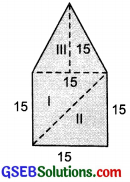Area of the triangle = $$\frac{1}{2}$$ × 15 × 15 = $$\frac{225}{2}$$ m2
= 112.5 + 225 = 337.5 m2
∴ Area of the pentagonal shape
= 112.5 + 225 = 3337.5 m2
Another way of finding the area of the pentagonal shape.
By splitting the pentagonal shape into 3 triang]es we have:
Area of traiangle I = $$\frac{1}{2}$$ × 15 × 15m2 = $$\frac{225}{2}$$ m2
Area of traiangle II = $$\frac{1}{2}$$ × 15 × 15m2 = $$\frac{225}{2}$$ m2
Area of traiangle III = $$\frac{1}{2}$$ × 15 × 15m2 = $$\frac{225}{2}$$ m2
∴ Area of the pentagonal shape
= $$\frac{225}{2}$$ m2 + $$\frac{225}{2}$$ m2 + $$\frac{225}{2}$$ m2 = 3337.5 m2Question 11.
Diagram of the adjacent picture frame has outer dimensions = 24 cm × 28 cm and inner dimensions 16cm × 20 cm. Find the area of each section of the frame, if the width of each section is same?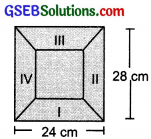Solution:
Area of trapezium I:
Parallel sides are 24 cm and 16 cm.
Height = $$\frac{28-20}{2}$$ = 4 cm
∴ Area = $$\frac{1}{2}$$ × [16 + 24] × 4 cm2
= 40 × 2cm2 = 80 cm2

Area of trapezium II:
Parallel sides are 20 cm and 28 cm
Height = $$\frac{24-16}{2}$$ = 4 cm
∴ Area = $$\frac{1}{2}$$ × [20 + 28] × 4 cm2
= 48 × 2 cm2 = 96 cm2

Area of trapezium III:
Area of trapezium III
= Area of trapezium III = Area of trapezium I = 80 cm2

Area of trapezium IV:
= Area of trapezium IV
= Area of trapezium = 96 cm2